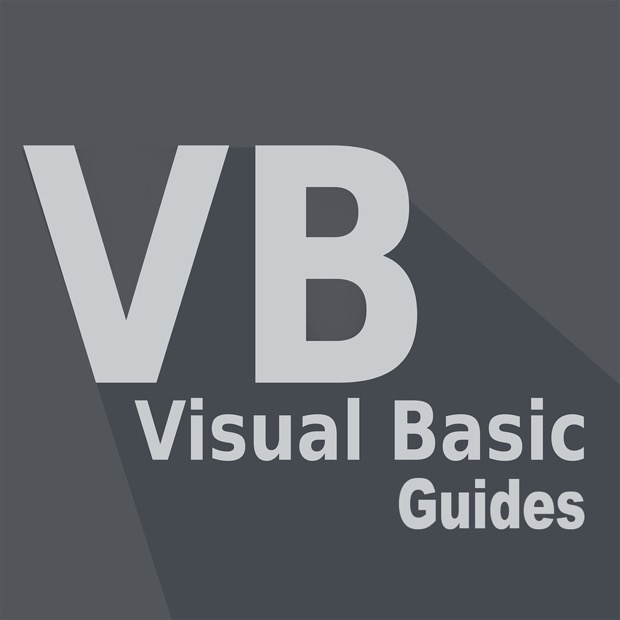\$5.99
Overview System Requirements Related

## Description

Like the BASIC programming language, Visual Basic was designed for an easy learning curve. Programmers can create both simple and complex GUI applications. This app has a series of over 250 Video tutorials that you can use to master the program. The app has many features designed to enhance and make easy your learning experience. You are able to customise the app in many ways including adding notes, ratings, favourites, re-ordering/grouping etc etc to make revisiting a previous lesson as easy as possible. Lessons include: Visual Basic Tutorial - 1 - What Is Visual Basic Visual Basic Tutorial - 16 - Conditional Operators Visual Basic Tutorial - 15 - Nested If Statements Visual Basic Tutorial - 12 - Creating A Basic Calculator Visual Basic Tutorial - 8 - Variables Visual Basic Tutorial - 6 - Comments And Whitespace Visual Basic Tutorial - 5 - Primitive Data Types Visual Basic Tutorial - 7 - Errors Visual Basic Tutorial - 14 - Else If And Else Visual Basic Tutorial - 11 - Getting User Input Visual Basic Tutorial - 9 - Math Operators Visual Basic Tutorial - 10 - More On Math Operators Visual Basic Tutorial - 17 - Logical Operators Visual Basic Tutorial - 20 - SubStrings Visual Basic Tutorial - 23 - Comparing Strings Visual Basic Tutorial - 22 - Replacing SubStrings Visual Basic Tutorial - 36 - Introduction To Windows Forms Visual Basic Tutorial - 19 - Get Length Of Strings Visual Basic Tutorial - 18 - Concatenate Strings Visual Basic Tutorial - 33 - More On Do Loops Visual Basic Tutorial - 21 - Formatting Strings Visual Basic Tutorial - 26 - For Next Loop Visual Basic Tutorial - 43 - Functions Visual Basic Tutorial - 40 - MessageBox Input Visual Basic Tutorial - 44 - ByVal Visual Basic Tutorial - 46 - Optional ByVal Visual Basic Tutorial - 47 - Coercion Visual Basic Tutorial - 45 - ByRef Visual Basic Tutorial - 27 - Step Operator Visual Basic Tutorial - 41 - Input Box Visual Basic Tutorial - 38 - ToolBox Visual Basic Tutorial - 42 - User Defined Subs Visual Basic Tutorial - 25 - Select Case Else Visual Basic Tutorial - 49 - Events Visual Basic Tutorial - 48 - Exit Subs Visual Basic Tutorial - 32 - Exit Do Loops Visual Basic Tutorial - 29 - Continue For Visual Basic Tutorial - 31 - Do While Loop Visual Basic Tutorial - 28 - Exiting For Loop Visual Basic Tutorial - 2 - Installing The Visual Basic IDE Visual Basic Tutorial - 39 - MessageBoxes Visual Basic Tutorial - 34 - Nested Loops Visual Basic Tutorial - 24 - Select Case Visual Basic Tutorial - 4 - Hello World

Billie Applications

Billie Applications

#### Developed by

Billie Applications

6/14/2019

7.39 MB

For all ages

#### Installation

Get this app while signed in to your Microsoft account and install on up to ten Windows 10 devices.

#### Language supported

English (United States)